back to all posts

# Interview Preparation - Basic JavaScript — 3

#### by Nabendu Biswas / October 25th, 2018

##### Series: Interview-webdev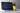Welcome to part-3 of the series. Let’s start with where we left.

Question 12- What is the difference between call, bind and apply?

Answer-call, bind and apply are used in calling a function, with some Object. You can think them of doing something like below.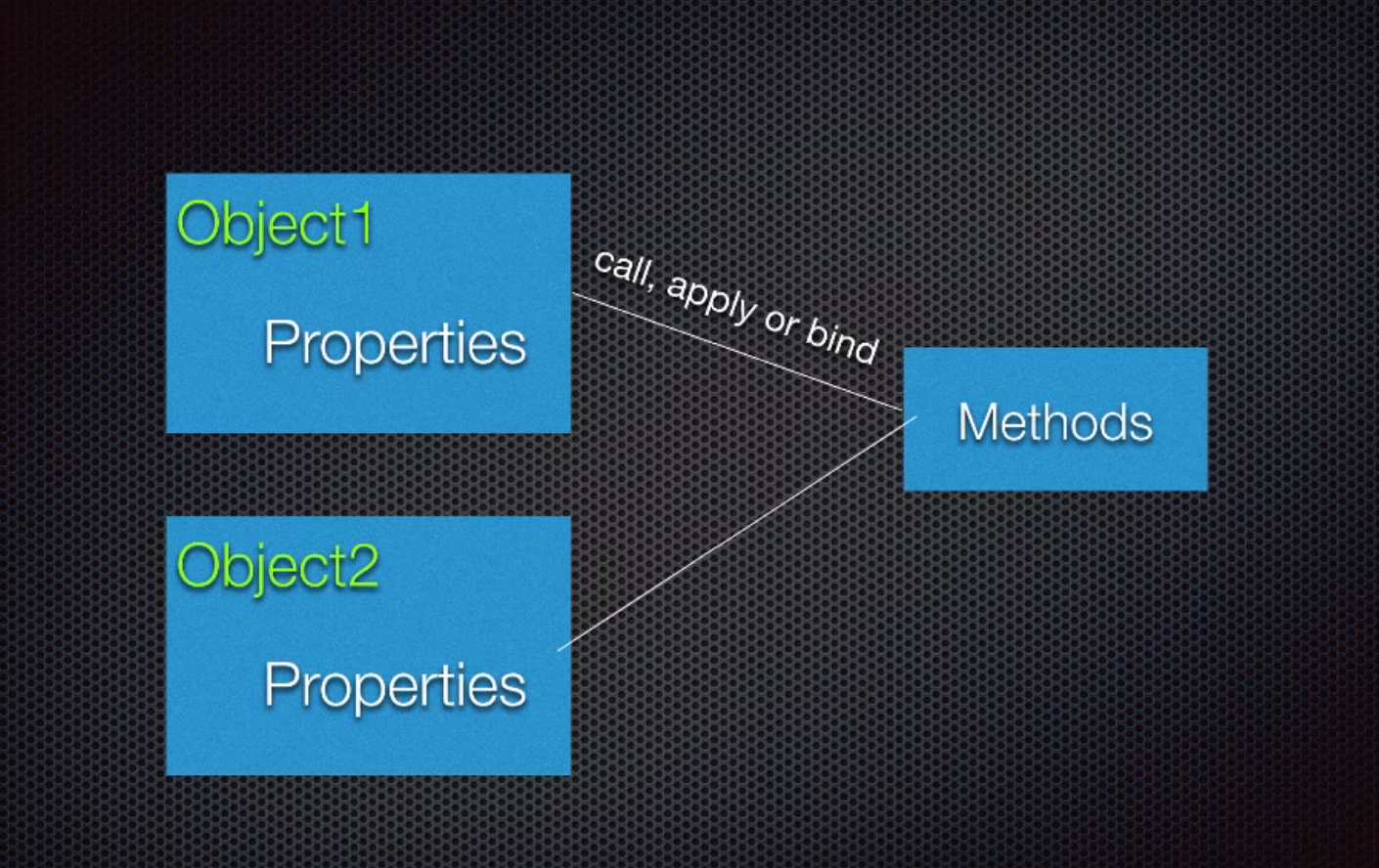call, bind and apply

### call

Let’s say that we have an object called obj. It only has one property called num, which has a value of 3. Let’s also make a function called addNumbers.

``````    let obj = {num: 3};
let addNumbers = function(a, b, c){
console.log(this);
return this.num + a + b + c;
};
``````

Now, in addNumbers we have this.num. But how do we pass the value obj.num to it. We need to pass it a context, which means the value of “this”. We will do this my call method by passing a first argument as obj, so the “this” is the obj now.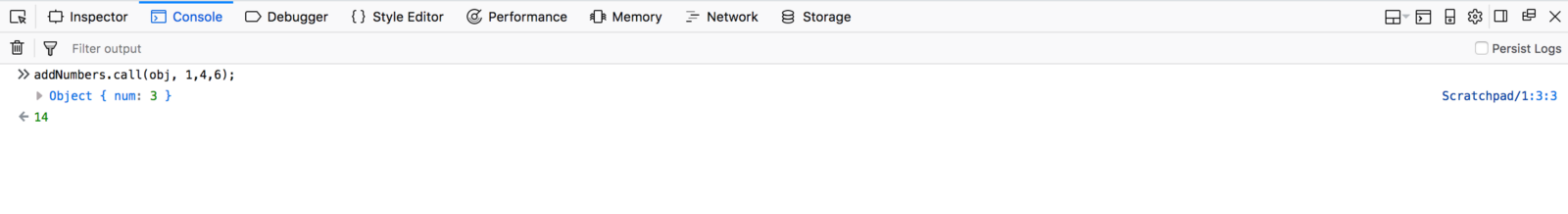call explained

### apply

It is totally similar to call, but the only difference is that we can pass array as the second argument. We will use the same code for apply also.

``````    let obj = {num: 3};
let addNumbers = function(a, b, c){
console.log(this);
return this.num + a + b + c;
};
``````

But now we have an array, to be passed as second argument.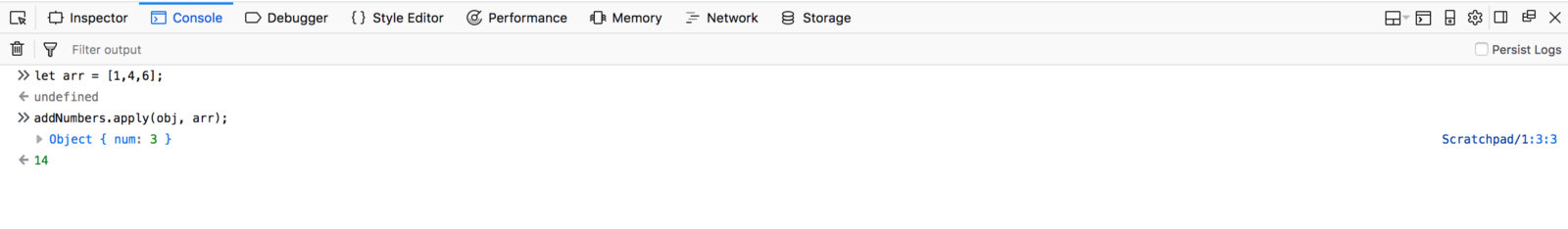apply explained

### bind

Bind works in a bit different way then call and apply. It works by returning a copy of the function. So, we will use the same code.

``````    let obj = {num: 3};
let addNumbers = function(a, b, c){
console.log(this);
return this.num + a + b + c;
};
``````

We will take the returned function in bindFunc and then execute it in the next line.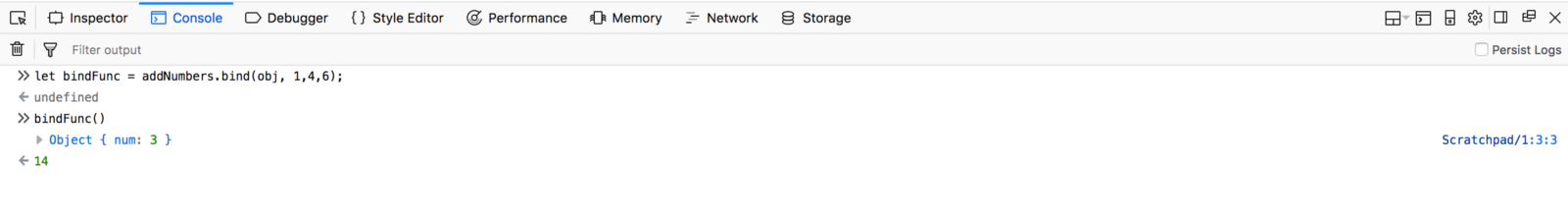bind explained

Question 13- Tell some practical applications of call, bind and apply?

Answer-We can use call to change an array like object to array. This is generally required as arrays have a lot of methods like map, reduce, filter which is required for manipulation of data. For example to convert the arguments array like object into array.

Consider the below example, in which we console log arguments. If we expand it in dev console, it shows its not and array and it’s proto doesn’t have array functionalities.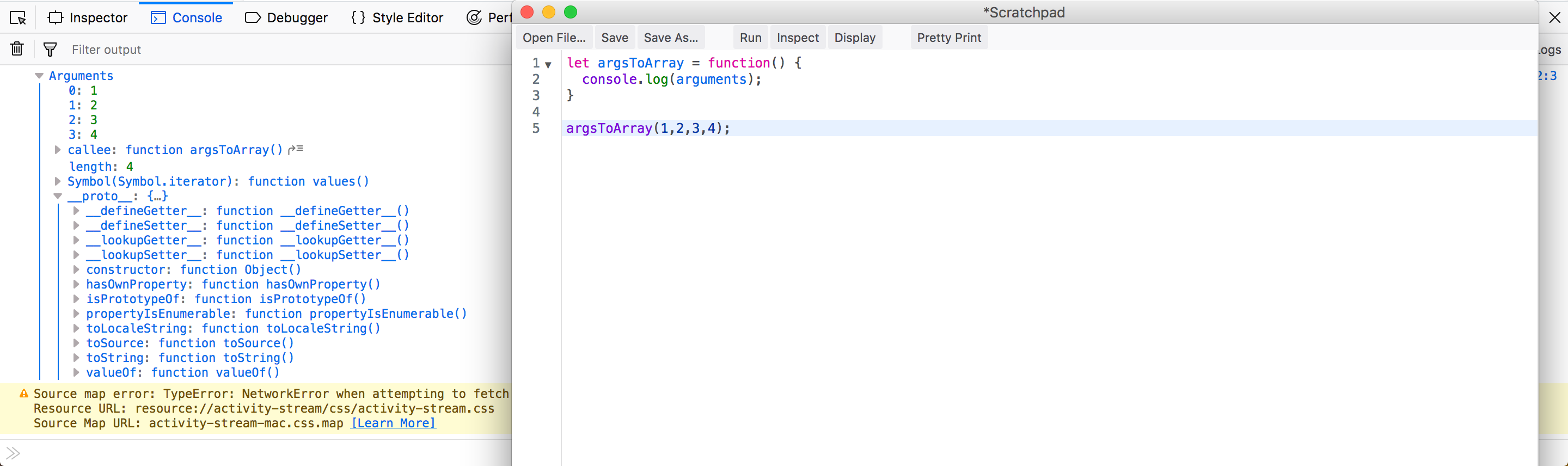The arguments object

Now to convert it we will take an empty array and use it’s slice method. Then call it through call, by passing the argument Object. Now it is converted into array and the proto have all array functionalities.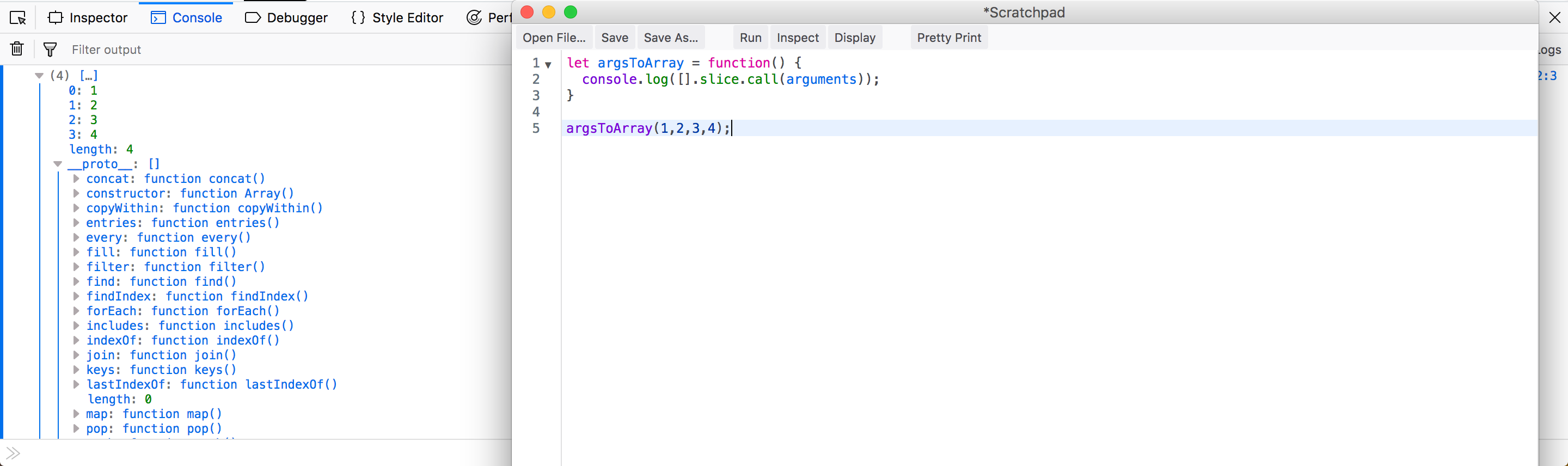The arguments object converted to array

The second application of call is in inheritance , using constructor functions. Using ES6 classes we have super() available in child class, but it cannot be done using traditional inheritance.

Consider the below example. Here in the Cat function, we called it’s parent Mammal using call method with “this” of Cat.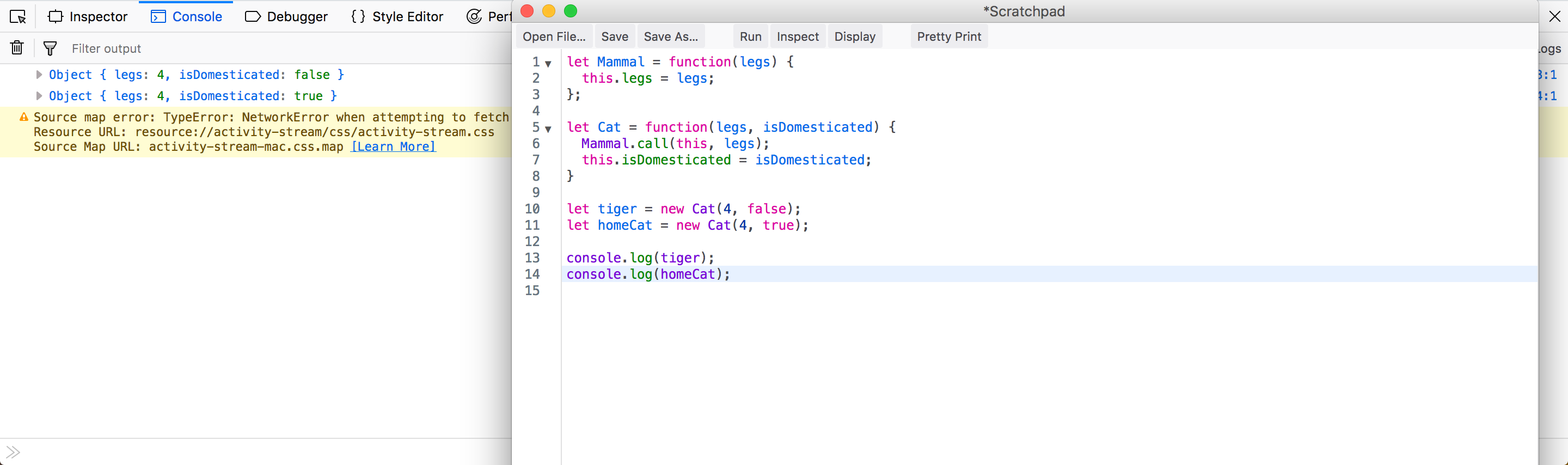*call in inheritance*

One of the practical use of apply is to pass an array to a function, which expects arguments only.

Consider the case of Math.min, which gives the minimum of the arguments passed. So, now to pass an array like in below code, we convert it through apply. Note the first argument is null because we are not passing any Object for “this” binding.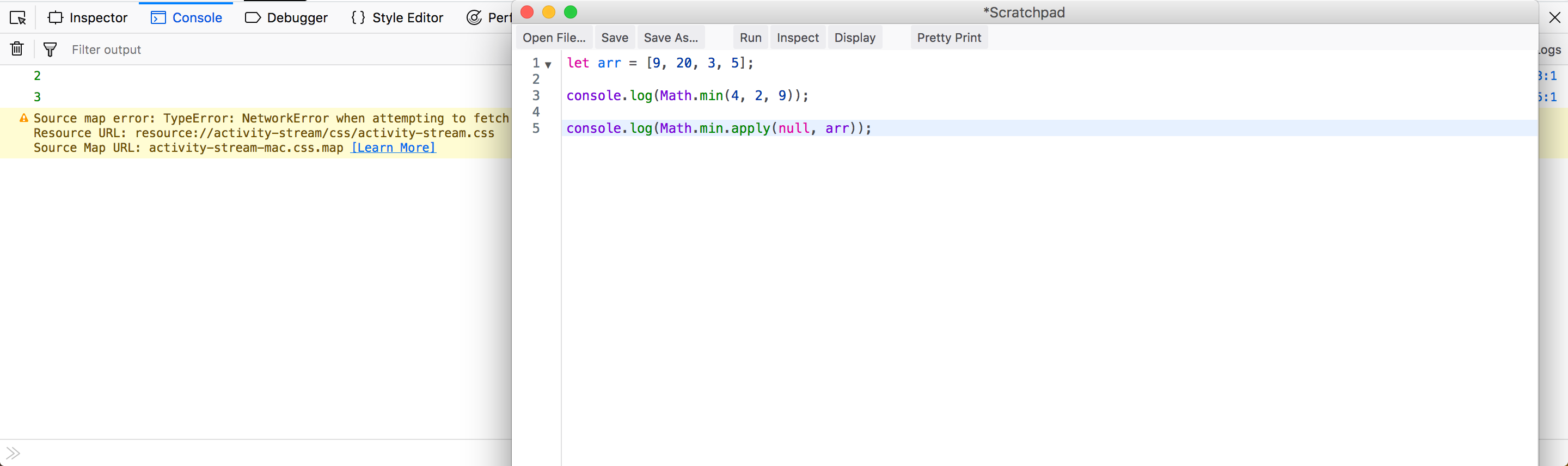apply use in Math.min

One of the practical use of bind is in React. In React whenever we call a function from render(), we have to bind it’s “this” in constructor.

Consider the below code. We bind the “this” of the event handler to the component instance in the constructor, we can pass it as a callback without worrying about it losing its context.

``````    class Foo extends React.Component{
constructor( props ){
super( props );
this.state = {
text: ''
}

this.handleChange = this.handleChange.bind(this);
}

handleChange(event){
this.setState({
text: event.target.value
})
}

render(){
return (
<input type="text" value={this.state.text}
onChange={this.handleChange} />
);
}
}

ReactDOM.render(
<Foo />,
document.getElementById("app")
);
``````

Note: Details of the above scenario in my article on “Demystifying this in JavaScript and Reacthere.

Question 14- How to remove duplicates from array?

Answer-There are many different ways to remove duplicates from an array.

### Solution 1

We can create another empty array and then traverse through the original array. We will then check if new array consists of the current element from original array. If it is not there then we will push it.

``````    let arr = [1, 2, 3, 5, 1 ,2 ,5];

let b = [];
for(let i=0; i<arr.length; i++) {
if(!b.includes(arr[i]))
b.push(arr[i]);
}

console.log(b);
//Output - [1, 2, 3, 5]
``````

### Solution 2

We can use a for..of loop to iterate over the original array and store it as element: true in an Object. Then we can get the array by Object.keys(obj)

``````    let arr = [1, 2, 3, 5, 1 ,2 ,5];

let obj = {};

for(let i of arr) {
obj[i] = true;
}

console.log(obj); // { 1: true, 2: true, 3: true, 5: true }

console.log(Object.keys(obj));
//Output- [ "1", "2", "3", "5" ]
``````

### Solution 3

We can use the new ES6 data structure Set. They have properties to only store unique values. But it returns an Set, which is not an array. So, to convert it into an array we use the Spread operator

``````    let arr = [1, 2, 3, 5, 1 ,2 ,5];

console.log(new Set(arr)); //Set(4) [ 1, 2, 3, 5 ]

console.log([... new Set(arr)]);
//Output - Array(4) [ 1, 2, 3, 5 ]
``````

Question 15- What is the difference between for..in and for..of loops?

Answer-The for..in loop is used to iterate over properties of an Objects. In JavaScript Arrays are also Objects and each characters in an string have an index. So, we can use them also to iterate over Arrays and Strings.

``````    //Object example
const obj = {
a: 1,
b: 2,
c: 3,
d: 4
}

for (const key in obj) {
console.log( obj[key] )
}
// Output: 1 2 3 4

//Array example
const array = ['a', 'b', 'c', 'd'];

for (const index in array) {
console.log(array[index])
}
// Output: a b c d

//String example
const string = 'Web Developer';

for (const index in string) {
console.log(string[index])
}
//Output: W e b D e v e l o p e r
``````

The for..of loop was introduced in ES6 and is used to iterate over Objects that have [Symbol.iterator] property. This includes array, strings and NodeList. They cannot be used to iterate over Objects are not iterable.

If we check the proto of an array, we can find the [Symbol.iterator] property.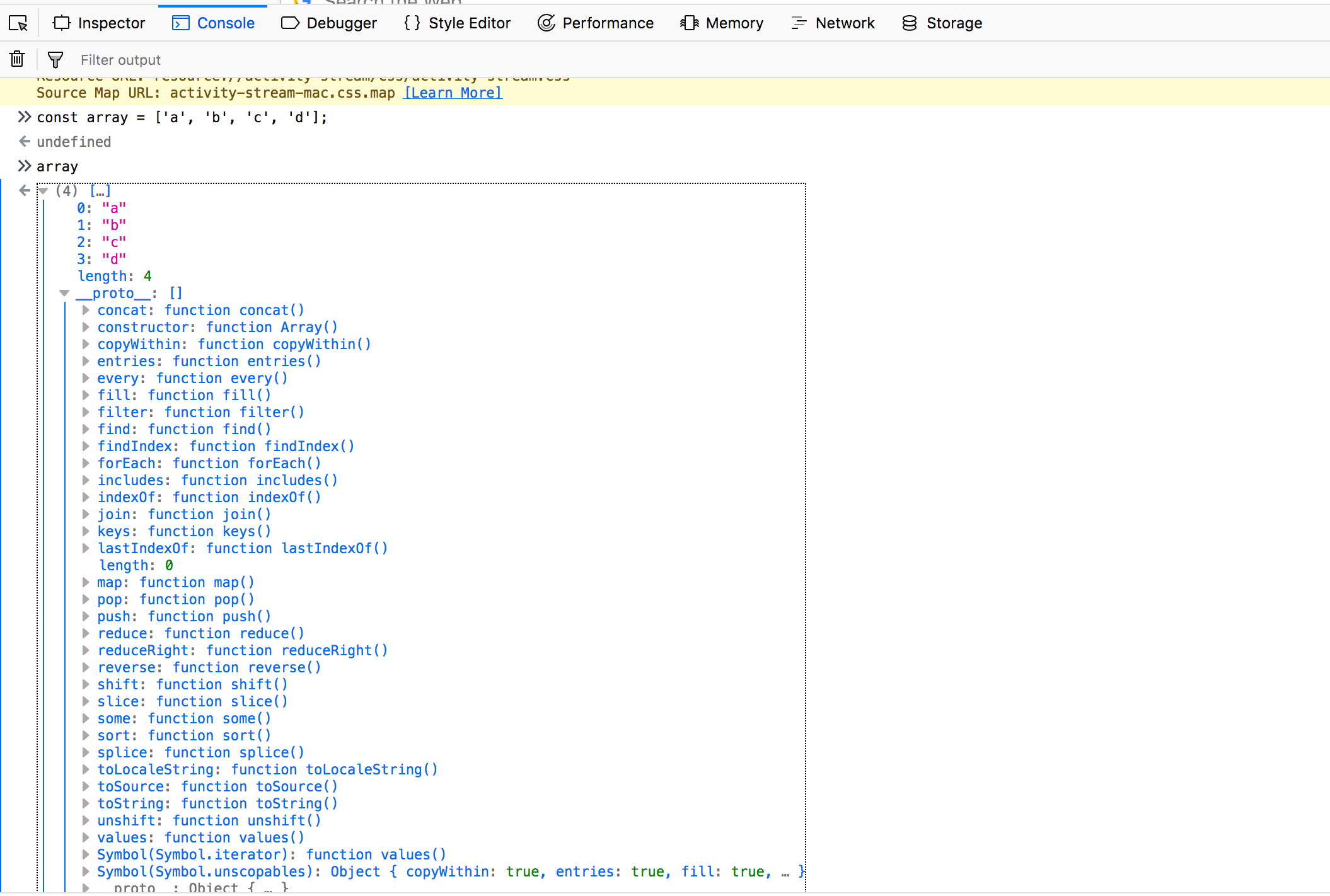[Symbol.iterator] in array

But it is not the case with Objects as it is not iterable.No [Symbol.iterator] in object

``````    //Array example
const array = ['a', 'b', 'c', 'd'];

for (const item of array) {
console.log(item)
}
// Output: a b c d

//String example
const string = 'Web Developer';

for (const character of string) {
console.log(character)
}
//Output: W e b D e v e l o p e r

//NodeList example
const elements = document.querySelectorAll('.foo');

for (const element of elements) {